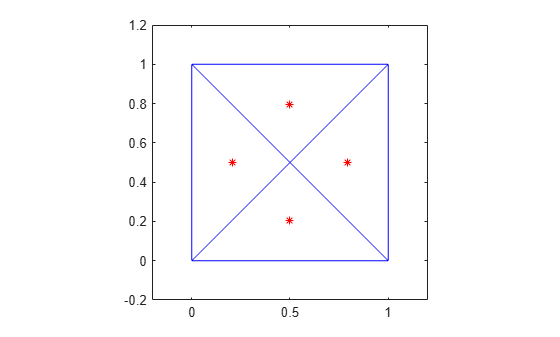# incenter

Incenter of triangulation elements

## Syntax

``C = incenter(TR)``
``C = incenter(TR,ID)``
``````[C,r] = incenter(___)``````

## Description

example

````C = incenter(TR)` returns the coordinates of the incenters of each triangle or tetrahedron in the triangulation `TR`.```

example

````C = incenter(TR,ID)` returns the coordinates of the incenter of each triangle or tetrahedron specified by `ID`. The identification numbers of the triangles or tetrahedra in `TR` are the corresponding row numbers of the property `TR.ConnectivityList`.```

example

``````[C,r] = incenter(___)``` also returns the radii of the inscribed circles or spheres.```

## Examples

collapse all

Create a 2-D Delaunay triangulation.

```x = [0 1 1 0 0.5]'; y = [0 0 1 1 0.5]'; TR = delaunayTriangulation(x,y);```

Compute the incenters of the triangles.

`C = incenter(TR);`

Plot the triangles and incenters.

```triplot(TR) axis equal axis([-0.2 1.2 -0.2 1.2]) hold on plot(C(:,1),C(:,2),'*r') ```Load a 3-D triangulation.

`load tetmesh`

Calculate the incenter coordinates of the first five tetrahedra in the triangulation, in addition to the radii of their inscribed spheres.

```TR = triangulation(tet,X); [C,r] = incenter(TR,[1:5]')```
```C = 5×3 -6.1083 -31.0234 8.1439 -2.1439 -31.0283 5.8742 -1.9555 -31.9463 7.4112 -4.3019 -30.8460 10.5169 -3.1596 -29.3642 6.1851 ```
```r = 5×1 0.7528 0.9125 0.8430 0.6997 0.7558 ```

## Input Arguments

collapse all

Triangulation representation, specified as a scalar `triangulation` or `delaunayTriangulation` object.

Data Types: `triangulation` | `delaunayTriangulation`

Triangle or tetrahedron IDs, specified as a scalar or a column vector whose elements each correspond to a single triangle or tetrahedron in the triangulation object. The identification number of each triangle or tetrahedron is the corresponding row number of the `ConnectivityList` property.

Data Types: `double`

## Output Arguments

collapse all

Incenters, returned as a matrix whose rows contain the coordinates of an incenter.

Data Types: `double`

Radii of the inscribed circles or spheres, returned as a vector.

Data Types: `double`

## Version History

Introduced in R2013a